Function

Why use pure functions?

• If a function is used many times over it can run 15 times faster if it's implemented as a pure function.
• Programming with pure functions is often more concise.
For example the following line uses a pure function in Select to return all elements of data with a magnitude less than 50.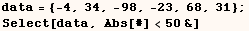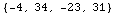The following line also returns all elements of data with a magnitude less than 50.  This version doesn't use pure functions. Notice the version using a pure function is more concise but seems cryptic until you get used to pure functions.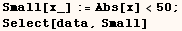Before looking at examples below it might help to clear definitions from all global symbols with the next cell.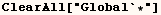Pure functions that use only one argument

The next cell defines a function (f) which returns 3 plus it's argument.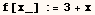In the next cell I give four different ways to define a pure function (g) which returns 3 plus it's first argument. The expression used to define the function varies depending which version is used, but as far as I can tell there is no meaningful difference between the different versions.
The Symbol (#) is short hand for Slot and it refers to the first argument given to a pure function.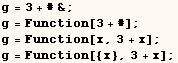In the next cell we see f[t] returns the same thing as g[t].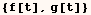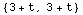Functions (f) and (g) don't always do the same thing.  Function (f) is only defined when given one argument, but the pure function (g) is defined when given one or more arguments.  The pure function (g) uses the first argument and ignores all other arguments.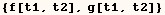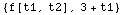Pure functions that use an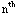argument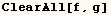In the next cell I define a function (f) that takes three arguments.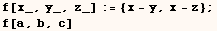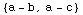In the next cell I give three ways to define a pure function that does essentially the same thing as (f) in the previous cell.  The FullForm of (#n)  is Slot[n], and we use (#2, and  #3) refer to the second and third arguments of a pure function. The third way of defining the pure function results in differnt form of storing the functions definition that the first two solutions, but as far as I can tell there is no meaningful difference between the different versions.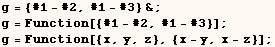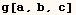Notice the previous definition for (g) will complain if it's given an insufficient number of arguments.  Because of this you shouldn't use pure functions to define a function an end user will use.  However, pure functions are a good choice for private functions in a package since the end user will not be using the private functions.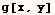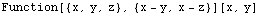Notice it's perfectly legal for a pure function to ignore some arguments before theargument.  To demonstrate this point I define (g) below which returns the sum of it's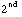and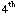arguments. In this case all other arguments are ignored.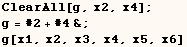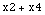Pure functions that use an arbitrary number of argumentsThe following cell defines a function (f) that puts all it's arguments in a list and squares each element.  The squaring operation is mapped to each element because Power has the Listable attribute, but that's another subject.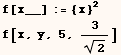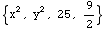In the next cell I give two ways to define a pure function that puts all its elements in a list and square each element.  The FullForm of ## is SlotSequence and is used to refer to all the arguments passed to a pure function.  The two styles used here result in exactly the same definition for the function.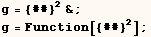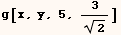Use of ##nThe next cell defines a pure function (g) that puts all it's arguments starting with the third argument in a list and squares each element. The FullForm of  (##3)  is SlotSequence and it refers to all arguments of a pure function from the third argument on.  The two styles used here result in exactly the same definition for the function.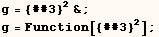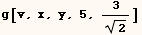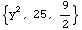Earlier I demonstrated that Mathematica will complain if a pure function uses #3 and is given less than three arguments.  So it's curious that Mathematica doesn't complain below when (g) tries to use ##3, but is given only two arguments.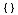Pure functions with one attributeIn the next cell I define a function (f) that returns True if it's argument is a Symbol before evaluating and otherwise returns False.  Programming this function is slightly tricky because evaluation is prevented in two places.  First we give (f) the HoldAll attribute to prevent it's argument from evaluating before using the definition of (f).  In addition we use Unevaluated[x] to prevent the argument (x) from evaluating before getting it's head.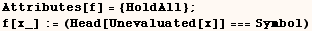In the next cell we see f[t] returns True even when (t) evaluates to a number rather than a symbol.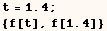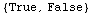In the next cell I give four ways to define a pure function (f) that does essentially the same thing as (f) defined above.In the next cell we see (g) does the same thing as (f).  I have found useful applications for this pure function.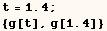Pure functions with multiple attributesThe next cell defines a function (f) which prevents it's arguments from evaluating, puts the arguments in canonical order, converts the arguments to strings and concatenates the sorted strings with another string.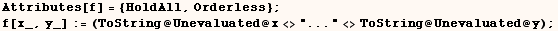In the next cell we see (f) in action.  You will find f[t,s] returns the same thing as f[s,t].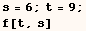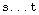The next cell defines a pure function (g) that does essentially the same thing as (f) above.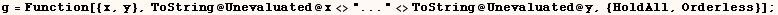In the next cell we see (g) in action.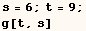Pure functions that use no arguments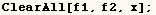(h&) is a pure function with no arguments which always returns (h). An occasion where this is needed is in the PolygonTessellation package available at http://library.wolfram.com/packages/. This package includes a function called TessellatePolygon3D with a WindingRule option. The WindingRule setting should be a function that returns True or False. A typical setting of this option is WindingRule→OddQ, but in some cases you want a test that always comes out True. In that case the setting WindingRule→(True &) should be used.

Often times the ButtonFunction for buttons is a pure function with no arguments.  I give such an example in my section on ButtonProgramming.

Consider f1, f2 in the next cell. Here the function f1 returns True no matter what argument(s) it has, and f2 returns a random number between 0 and 1 no matter what argument(s) it has.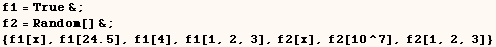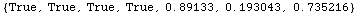Keep in mind (f1) isn't True it's a function that always returns True and that explains why the first element of the next list is False.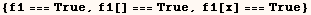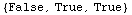Use of  #0  (rarely useful)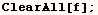When used in a pure function (#0) represents the pure function itself.  I can't imagine when this would be useful, but I demonstrate it in with the pure function (f) in the next cell.  This pure function prints (The second argument is ...) and returns the entire pure function as an expression.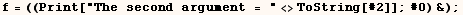In the next cell we see (f) in action.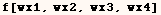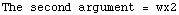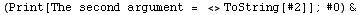Also read  "Further Examples" at the end of the  Function  documentation in the Help Browser.

Created by Mathematica  (May 16, 2004)

Back to Ted’s Tricks index page